Advertisement Remove all ads

# Resistances R1, R2, R3 and R4 Are Connected as Shown in the Figure. S1 and S2 Are Two Keys. Discuss the Current Flowing in the Circuit in the Following Cases. - Science and Technology

Answer in Brief
Diagram

Resistances R1, R2, R3 and R4 are connected as shown in the figure. S1 and S2 are two keys. Discuss the current flowing in the circuit in the following cases.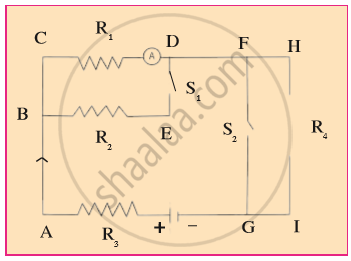a. Both S1 and S2 are closed.
b. Both S1 and S2 are open.
c.  S1 is closed but S2 is open.

Advertisement Remove all ads

#### Solution

a. The circuit for this case is shown below.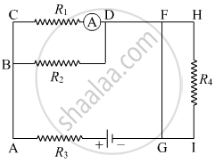Here, R1 and R2 are in parallel. Their effective resistance is
R' = (R_1 xx R_2)/(R_1 + R_2)
Also, R4 is in parallel with a conductor of 0 resistance. Their equivalent resistance is therefore 0. The given circuit thus becomes as shown below.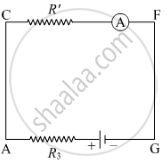Now , R and R_3 are in series . Thus , the quivalent resistance of the circuit is
R_(eq) = (R_1 xx R_2)/(R_1 + R_2) + R_3 = (R_1R_2 + R_1R_3 + R_3R_2)/(R_1+R_2)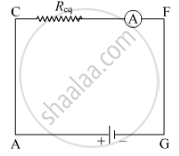Hence, the current flowing in the circuit is
I = V/((R_1R_2 + R_1R_3 + R_3R_2)/(R_1 + R_2)) = (V(R_1 + R_2))/(R_1R_2 + R_1R_3 + R_3R_2)
where , V is the potential difference of the battery .
b.The circuit for this case is shown below.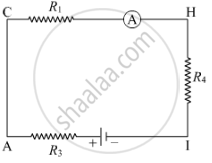Here, R1, R4 and R3 are in series. Therefore, the equivalent resistance of the circuit is
R_(eq) = R_1 + R_2 + R_3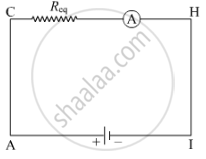Hence, the current flowing in the circuit is
I = V/R_(eq) = V/(R_1 + R_2 + R_3)
where, V is the potential difference of the battery.
C.The circuit for this case is shown below.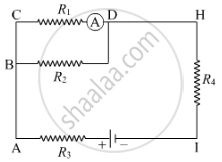Here , R1 and R2 are in parallel . their effective resistance is
R' = (R_1 xx R_2)/(R_1 + R_2)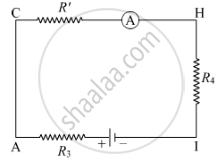Now , R3 , R and R4 are in series . Thus , the equivalent resistance of the circuit is
R_(eq) = (R_1 xx R_2)/(R_1 +R_2) + R_3 + R_4 = (R_1R_2 + R_1R_3 + R_3R_2 + R_1R_4 + R_4R_2)/(R_1 + R_2)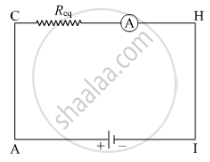Hence , the current flowing in the circuit is
I = (V/(R_1R_2 + R_1R_3 + R_3R_2 + R_1R_4 + R_4R_2))/(R_1 + R_2) = (V(R_1 + R_2))/(R_1R_2 + R_1R_3 + R_3R_2 + R_1R_4 + R_4R_2)
where , V is the potential difference of the battery.

Is there an error in this question or solution?
Advertisement Remove all ads

#### APPEARS IN

Balbharati Science and Technology 9th Standard Maharashtra State Board
Chapter 3 Current Electricity
Exercise | Q 7 | Page 45
Advertisement Remove all ads
Advertisement Remove all ads
Share
Notifications

View all notifications

Forgot password?